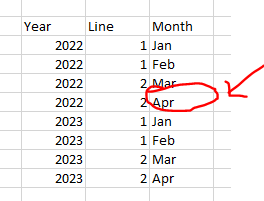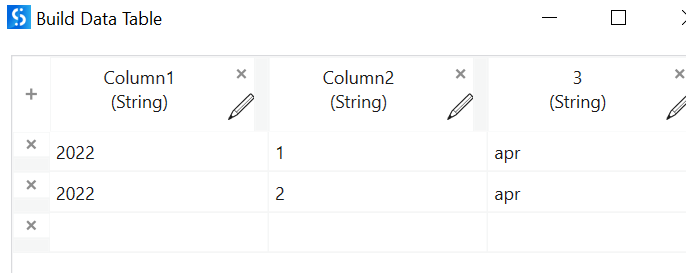# How to get row index based on location?

Dear community,

I am having trouble identifying the row index based on this cell (red circle)?

Can someone help me solve this problem?Use find/Replace in excel it would give you the exact details

cheers

Hi @Anil_G

i dont find this activies in my UIpath.

Is it this additonal package need to be install?

@JustZaffan

Are you on modern experience s the excel package updates?

cheers

Hi,

How about to use LookUpRange activity as the following?

This returns address string. We can extract row number from it using the following expression.

``````System.Text.RegularExpressions.Regex.Match(strAddress,"\d+\$").Value
``````

OR

``````CInt(System.Text.RegularExpressions.Regex.Match(strAddress,"\d+\$").Value)
``````

Regards,

nope. i just realised that i am not using the modern experience.

But when i enable it, i found it.

1 Like

Can we define the keyword?

Because in my case, i have 3 keyword which is 2022,2 and apr.

If you want to find the index only then youc an try thsi as well

`dt.rows.indexOf(dt.AsEnumerable.Where(function(x) x(0).ToString.Equals("2022") AND x(1).ToString.Equals("2") AND x(2).ToString.ToLower.Equals("apr")).FirstOrDefault)`

This will directly give you the index of matched row

you can change the conditions as per need

cheers

Hi @Anil_G ,

for find/replace value activity,

can we specify the value to find based on conditions?

for example, i have 3 condition.

let say it passed this 3 condition, it will select that cell.

Please see my previous comment …That way you can give multiple conditions

copying the same here

`dt.rows.indexOf(dt.AsEnumerable.Where(function(x) x(0).ToString.Equals("2022") AND x(1).ToString.Equals("2") AND x(2).ToString.ToLower.Equals("apr")).FirstOrDefault)`

dt is the datatable you have data in

Output is an interger directly assign the value to a integer variable

cheers

Hi,

FYI, another approach

We can use ForEachExcelRow for it in modern excel as the following.

Regards,

hi @anil

i already try using method but it return me -1.

i am not sure why it return that value

It might be because the values might have spaces after every tostring try including a trim and it should work

Or instead of equals use contains

cheers

@JustZaffan

Try this it worked

`dt.rows.indexOf(dt.AsEnumerable.Where(function(x) x(0).ToString.Contains("2022") AND x(1).ToString.Contains("2") AND x(2).ToString.ToLower.Contains("apr")).FirstOrDefault)`

Inputoutput

cheers

Just wondering

if the condition has 3 value to consider, we can also add “andalso” for the thrird condition

currentrow(“year”).tostring = “2022” and also currentrow(“month”).tostring = “april” currentrow(“line”).tostring = “1”

Yes, like the following.

``````currentrow("year").tostring = "2022" andalso currentrow("month").tostring = "april" AndAlso currentrow("line").tostring = "1"
``````

Regards,

it still return -1,

just to be clear

dt.rows.indexOf(dt.AsEnumerable.Where(function(x) x(0).ToString.Contains(“2022”) AND x(1).ToString.Contains(“2”) AND x(2).ToString.ToLower.Contains(“apr”)).FirstOrDefault)

is it x(0) in statement above refer to the first column?

Yes x(0) refers to the first column

index start from zero

You can as well give column names instead of index

cheers

i already try to run the work flow as per suggested by you.

it not works.

it just run through the whole row and end without any return current index

HI,

Can you share your worksheet? it’s no problem if dummy data.

Regards,